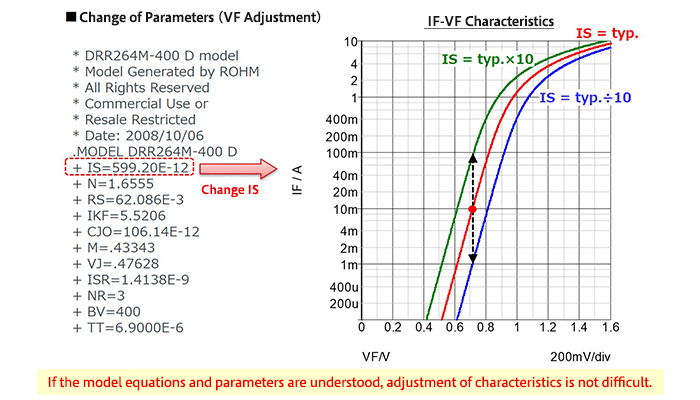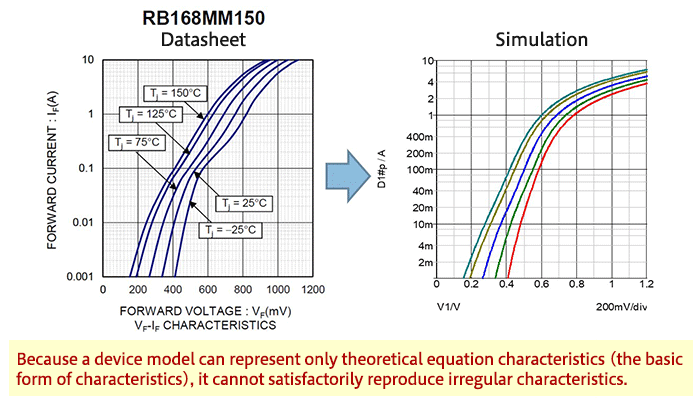Technical Information Site of Power Supply Design

2019.05.09 Simulation

# SPICE Device Models: Diode Example--Part 2

Foundations of Electronic Circuit Simulation

Following the previous article (Part 1), the explanation of SPICE device models was continued, using as an example a device model of a diode.

SPICE Device Models: Adjusting Parameters in Diode Device Model

In a device model, parameters are set, and so it is easy to see that if the settings in the model description are changed, these changes will be reflected in the simulation results. Below we present an example in which VF in the previously described diode device model has been adjusted.In order to adjust the diode VF, IS (the saturation current) is changed. The equation for IF in Part 1 is IF = IS*exp(Vd/N*Vt)-1), and from the equation we see that a change in IS is proportional to a change in IF. The graph shows simulation results for the IF-VF characteristic when the IS value is made 10 times greater, and reduced to 1/10 the previous value. The IF for VF = 0.7 V is increased and decreased by a factor of 10.

In order to understand the relationships between parameters and diode characteristics, the model equations and parameters must be understood.

Limits to SPICE Device Models

Device models essentially represent the characteristics of theoretical equations of the model. Hence characteristics that cannot be expressed by theoretical equations are not reflected in simulation results. Simulation results must be interpreted keeping this limitation in mind.

The graphs below show IF-VF characteristics of diodes different from those discussed above. On the left are typical characteristics of an actual diode as given by the datasheet; on the right are simulation results for the device model for this diode.It should be clear that, when comparing the linearity of IF-VF characteristics, simulation results are based on theoretical equations.

#### Key Points:

・There are two types of SPICE models: “device models” and “subcircuit models”.

・Characteristics can be adjusted by changing parameter values of device models.

・Device models are based on theoretical equations, and so simulation results are limited to the range that can be expressed by such equations (in some cases they may differ from actual behavior).